# A guitar string is 90 cm long and has a mass of 3.5 g. The distance...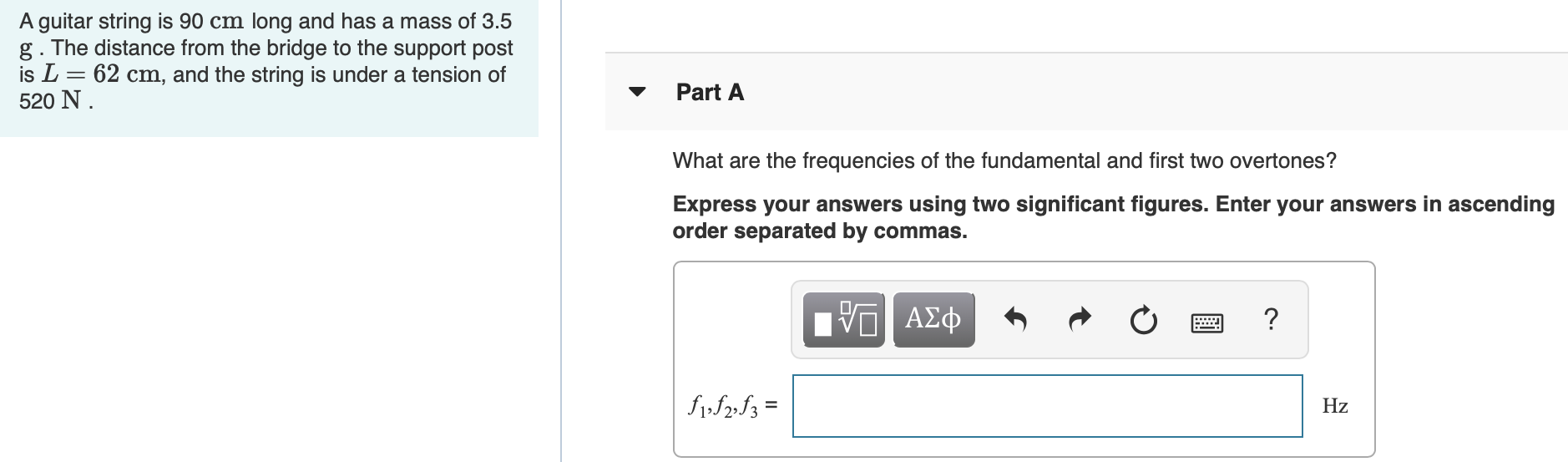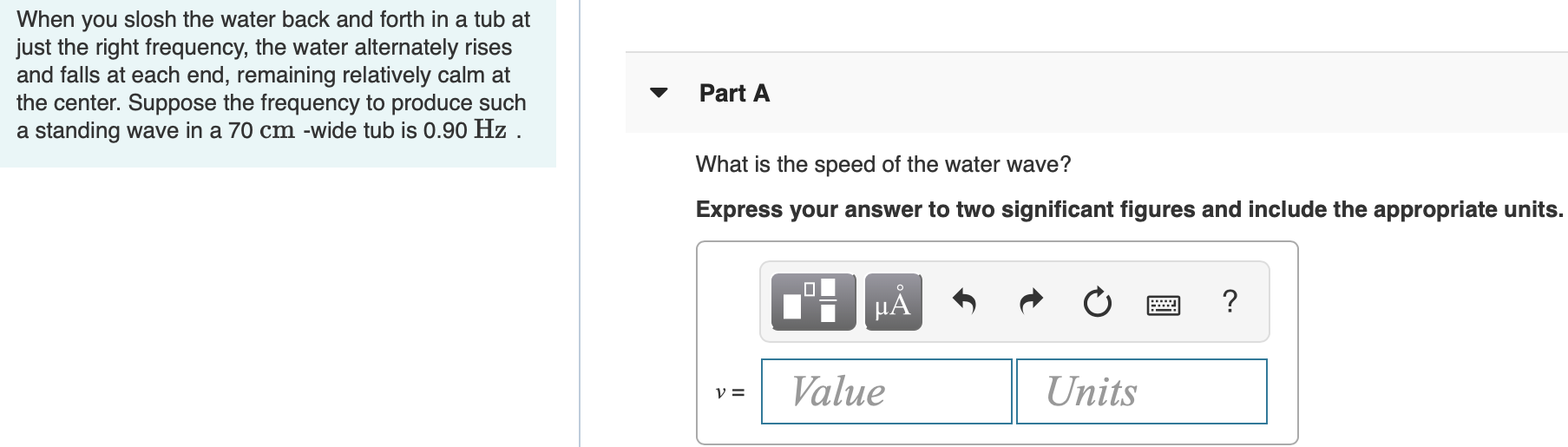A guitar string is 90 cm long and has a mass of 3.5 g. The distance from the bridge to the support post is L= 62 cm, and the string is under a tension of 520 N. Part A What are the frequencies of the fundamental and first two overtones? Express your answers using two significant figures. Enter your answers in ascending order separated by commas. ΟΙ ΑΣΦ ? \$1,12,13 = Hz
When you slosh the water back and forth in a tub at just the right frequency, the water alternately rises and falls at each end, remaining relatively calm at the center. Suppose the frequency to produce such a standing wave in a 70 cm -wide tub is 0.90 Hz . Part A What is the speed of the water wave? Express your answer to two significant figures and include the appropriate units. I H o ? VE Value Units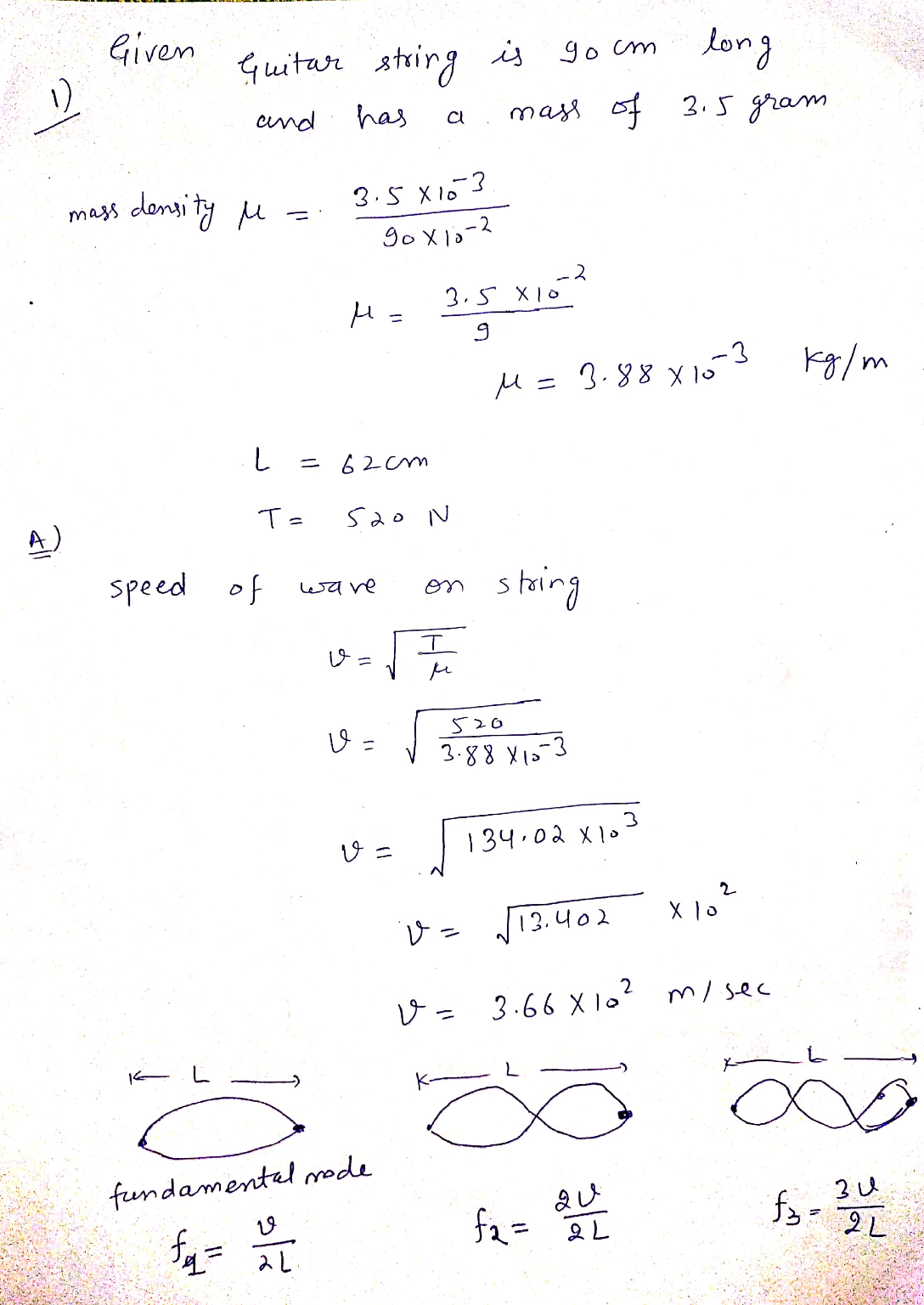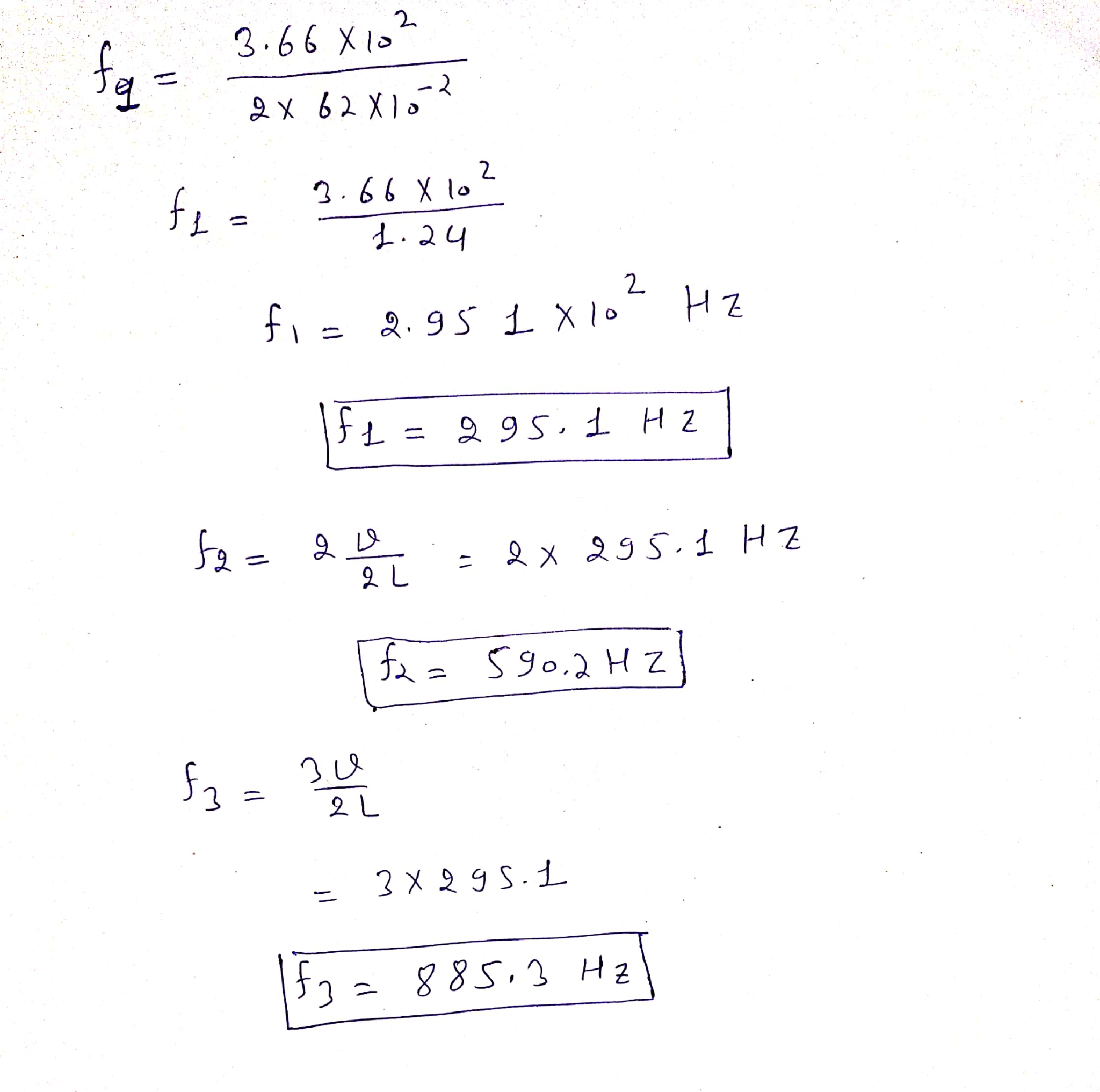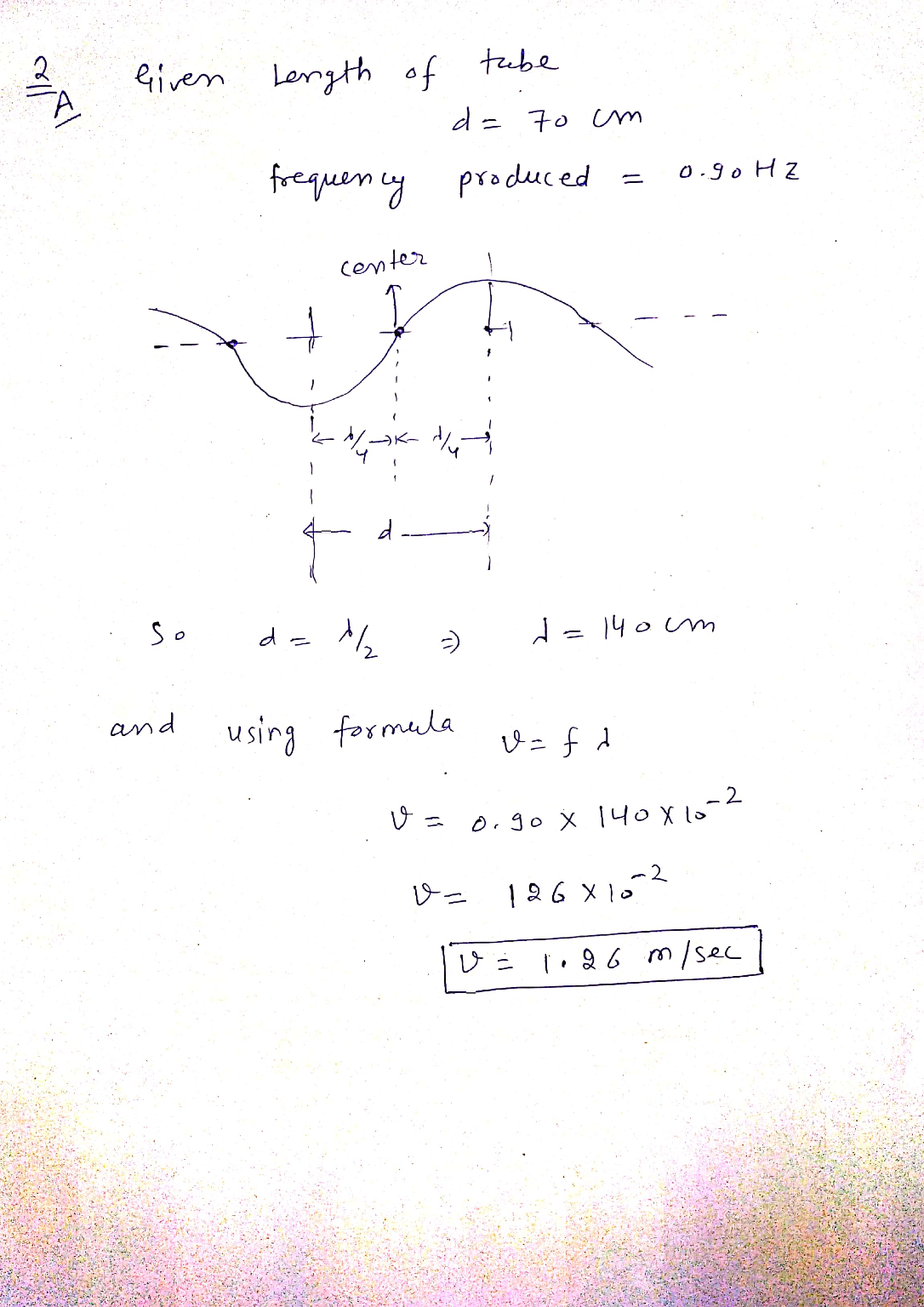##### Add Answer of: A guitar string is 90 cm long and has a mass of 3.5 g. The distance...
Similar Homework Help Questions
• ### A guitar string is 90 cm long and has a mass of 3.6 g. The distance...A guitar string is 90 cm long and has a mass of 3.6 g. The distance from the bridge to the support post is L 62 cm and the string is under a tension of 530 N. Part A What are the frequencies of the fundamental and first two overtones? Express your answers using two significant figures. Enter your answers in ascending order να ΑΣφ Hz Submt BssestAnse Provide Feedback Why VAIO SONY

• ### A guitar string is 90 cm long and has a mass of 3.6 g . The...

A guitar string is 90 cm long and has a mass of 3.6 g . The distance from the bridge to the support post is L=62cm, and the string is under a tension of 540 N . What are the frequencies of the fundamental and first two overtones? Express your answers using two significant figures. Enter your answers in ascending order separated by commas.

• ### When you slosh the water back and forth in a tub at just the right frequency,...

When you slosh the water back and forth in a tub at just the right frequency, the water alternately rises and falls at each end, remaining relatively calm at the center. Suppose the frequency to produce such a standing wave in a 65 cm -wide tub is 0.90 Hz. What is the speed of the water wave? Express your answer to two significant figures and include the appropriate units.

• ### The G string on a guitar is 59 cm long and has a fundamental frequency of...The G string on a guitar is 59 cm long and has a fundamental frequency of 196 Hz. A guitarist can play different notes by pushing the string against various trets, which changes the string's length. The fifth fret from the neck gives C (261.63 Hz) the sich fret gives Db (277.18 Hz). Part A How far apart are the fifth and sixth frets? Express your answer to two significant figures and include the appropriate units. HA ? AL =...

• ### Constants Part A A gutar string is 90 cm long and has a mass of 38...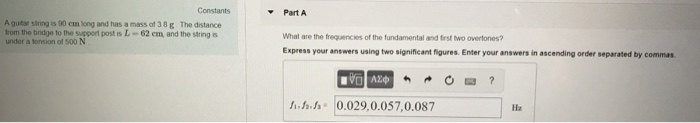Constants Part A A gutar string is 90 cm long and has a mass of 38 g The distance rom the bidge to the support post is L- 62 em, and the string under a tonsion of 500 N What are the frequencies of the fundamental and irst two overtones? Express your answers using two significant figures. Enter your answers in ascending order separated by commas んふか(0.029,0.057,0.087

• ### when you slosh the water back and forth in a tub at just the right frequency,...

when you slosh the water back and forth in a tub at just the right frequency, the water alternately rises and falls at each end, remaining relatively calm at the center. Suppose the frequency to produce such a standing wave in a 70 cm -wide tub is 0.75 Hz . Part A What is the speed of the water wave?

• ### <Ch 11 HW Problem 11.54 20 of 21 > Constants A guitar string is 90 cm...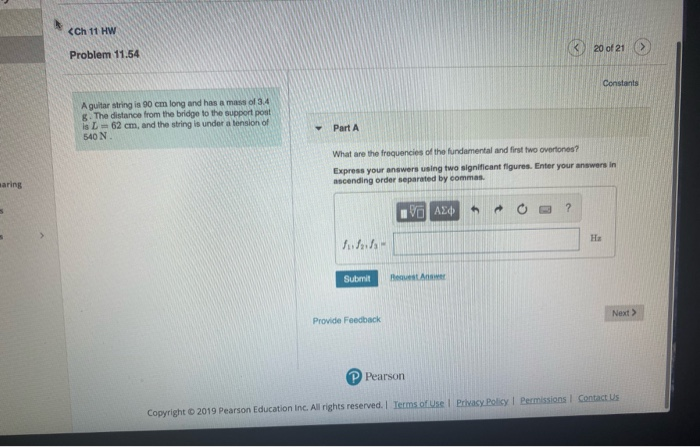<Ch 11 HW Problem 11.54 20 of 21 > Constants A guitar string is 90 cm long and has a mass of 3.4 8. The distance from the bridge to the support post is L=62 cm, and the string is under a tension of 540 N. Part A What are the frequencies of the fundamental and first two overtones? Express your answers using two significant figures. Enter your answers in ascending order separated by commas. aring 0 AED O2 ?...

• ### Problem 15.50 Constants I Periodic Table Part A A guitar string is 90.0 cm long and...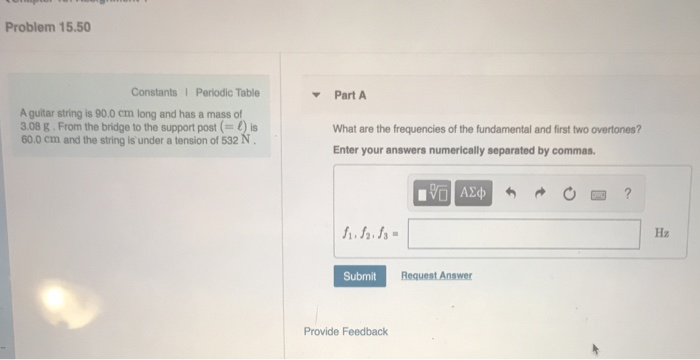Problem 15.50 Constants I Periodic Table Part A A guitar string is 90.0 cm long and has a mass of 3.08 g. From the bridge to the support post () is 60.0 cm and the string is' under a tension of 532 N What are the frequencies of the fundamental and first two overtones? Enter your answers numerically separated by commas. ν ΑΣφ 5 Hz Request Answer Submit Provide Feedback

• ### A string vibrates at its third-harmonic frequency Review Part A A string vibrates at its third-harmonic frequency. The amplitude at a point 42 cm from one end is half the maximum amplitude How long i...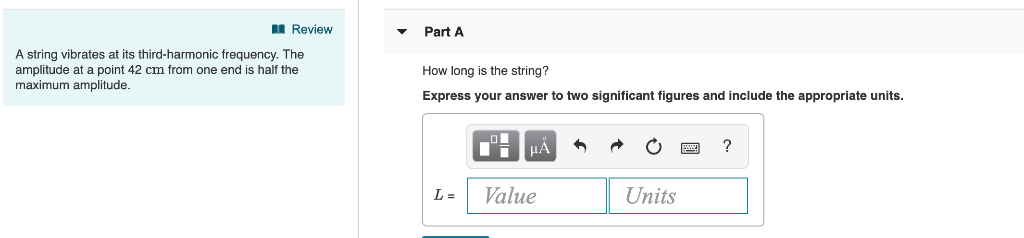A string vibrates at its third-harmonic frequency Review Part A A string vibrates at its third-harmonic frequency. The amplitude at a point 42 cm from one end is half the maximum amplitude How long is the string? Express your answer to two significant figures and include the appropriate units. Value Units Review Part A A metal wire under tension To vibrates at its fundamental frequency For what tension will the third-harmonic frequency be the same as the fundamental frequency at...

• ### Constants PartA An organ pipe is 118 cm long. The speed of sound in air is...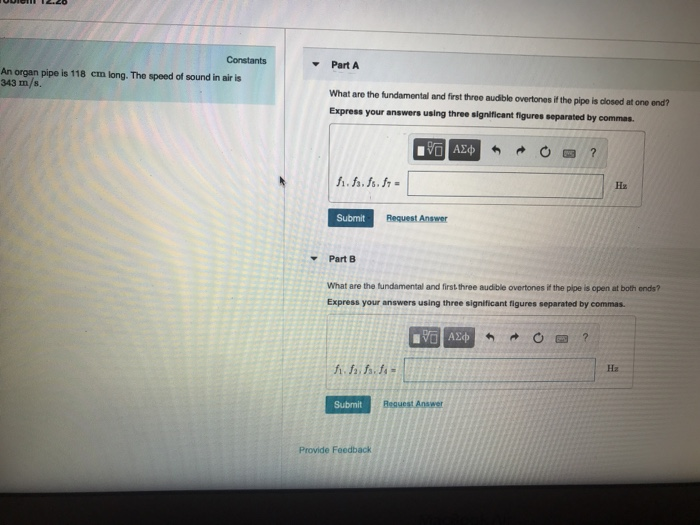Constants PartA An organ pipe is 118 cm long. The speed of sound in air is 343 m/s. What are the fundamental and first three audible overtones if the pipe is dlosed at one ond? Express your answers using three significant figures separated by commas Hz Submit Request Answer Part B What are the fundamental and first three audible overtones it the pipe is open at both ends? Express your answers using three significant figures separated by commas. Hz Submit...

Free Homework App